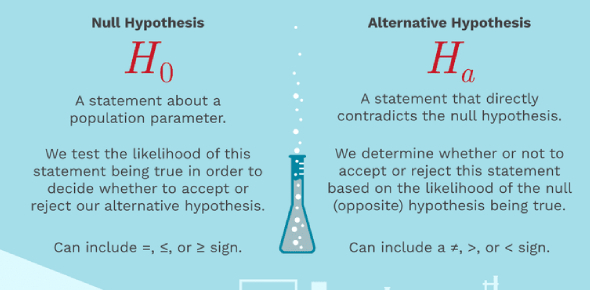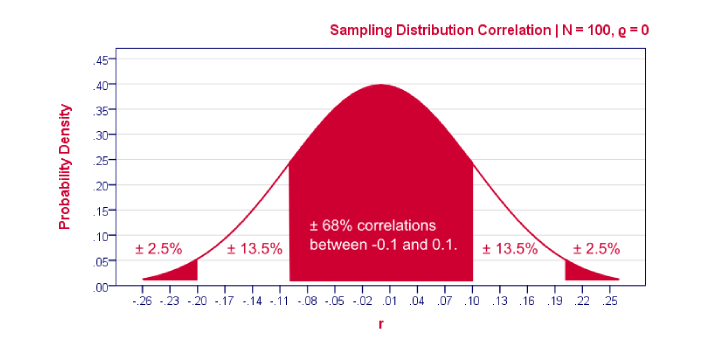What is the difference between Null and Alternative Hypothesis? - ProProfs DiscussTopicsMoreProductsMore+ Ask Question

# What is the difference between Null and Alternative Hypothesis?

Asked by I. Klose, Last updated: Mar 30, 2020

###Request 0FollowShareAnswer AnonymouslyAnswer LaterCopy Link#### Bobby Rickets

Bobby Rickets, Content Reviewer

The hypothesis which is to be tested is the null hypothesis, whereas the alternative hypothesis offers an alternative to the null hypothesis. The null hypothesis is a statement in which there is no relationship between two variables. An alternative hypothesis is a statement in which there is some statistical worth between two measured phenomena.Testing for the null hypothesis is indirect and implicit. Testing for the alternative hypothesis is direct and explicit. The null hypothesis is represented by H zero. The alternative hypothesis is one. The mathematical formulation for the null hypothesis is an equal sign. Testing for the alternative hypothesis is the unequal sign. With the null hypothesis, if there are no changes in opinions or actions, then no alteration is made. With the alternative hypothesis, if the alternative is accepted, then adjustments are made.#### j. Goodman

J. Goodman, Web Content Writer, San AntonioCreating a paper will not be complete without the use of the null and alternative hypothesis. These are both statements that you will use to prove a point. When you use the null hypothesis, you are showing that there is no relation between the different elements that are involved in the study.If you use the alternative hypothesis, you are showing that there is a relationship with the different elements that you are trying to study. The null hypothesis will usually be represented with H0 while the alternative hypothesis will be represented by placing H1. Take note that these two statements also use different formulas.#### H. Barnes

Subscribe to Pewdiepie

H. Barnes, Unemployed, Barnes, Atlanta

Both are statements. In a statement with a null hypothesis, there is no relationship between the two variables. A researcher will try to disprove this. In a statement with an alternative hypothesis, there is some significance between the two. A researcher will try to prove it. An alternative hypothesis will observe some effect, while a null hypothesis will not.Null hypothesis will not observe an effect, which is represented by H0. Alternative hypothesis will observe an effect, represented by H1. Each have a mathematical formula. The formula of a null hypothesis is an equal sign. The formula of an alternative hypothesis is a not equal sign.Search for Google imagesSelect a recommended image
Upload from your computerCancelSearch for Google imagesSelect a recommended image
Upload from your computerCancelSearch for Google imagesSelect a recommended image
Upload from your computerCancel